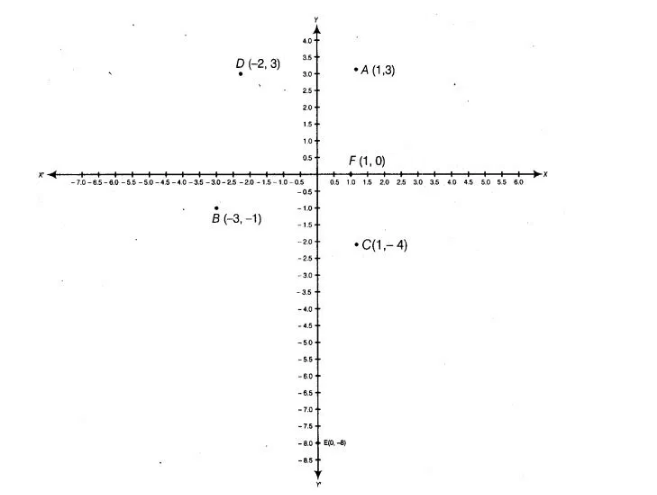# Taking 0.5 cm as 1 unit, plot the following points on`
Question:

Taking 0.5 cm as 1 unit, plot the following points on the graph paper A( 1, 3), 6(-3, -1,), C( 1, -4), D(-2, 3), E(0, -8) and F(1, 0).

Solution:

Here, in point 4(1, 3) both x and y-coordinates are positive, so it lies in I quadrant. In point 8(-3, -1),both x and y-coordinates are negative, so it lies in

III quadrant. In point C(1, -4), x-coordinate is positive and y-coordinate is negative, so it lies in IV quadrant.

In point D(-2, 3), x-coordinate is negative and y-coordinate is positive, so it lies in II quadrant.

In point E(0,-8) x-coordinate is zero, so it lies on Y-axis and in point F(1,0) y-coordinate is zero, so it lies on X-axis.On plotting the given points, we get the following graph.